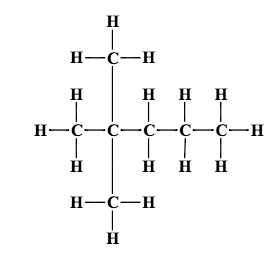# Problem: Convert the following structural formula into its condensed formula.

###### Problem Details

Convert the following structural formula into its condensed formula.What scientific concept do you need to know in order to solve this problem?

Our tutors have indicated that to solve this problem you will need to apply the Structural Formula concept. You can view video lessons to learn Structural Formula. Or if you need more Structural Formula practice, you can also practice Structural Formula practice problems.

What is the difficulty of this problem?

Our tutors rated the difficulty ofConvert the following structural formula into its condensed ...as low difficulty.

How long does this problem take to solve?

Our expert Chemistry tutor, Jules took 2 minutes and 28 seconds to solve this problem. You can follow their steps in the video explanation above.

What professor is this problem relevant for?

Based on our data, we think this problem is relevant for Professor Fisk's class at CU.